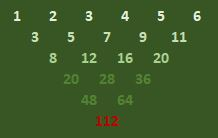# Problem 50063. Number Puzzles - 019

Borrowing the idea from Pascal Triangle, the following operation can be performed. Starting with an array of numbers from 1 to N (where N is greater than 1), the following upside-down triangle of numbers can be formed where starting from the second row, the number is determined by adding the two neighboring numbers in the previous row.Given N, determine the sum of all numbers in the triangle.

### Solution Stats

64.1% Correct | 35.9% Incorrect
Last Solution submitted on Oct 25, 2023

### Community Treasure Hunt

Find the treasures in MATLAB Central and discover how the community can help you!

Start Hunting!